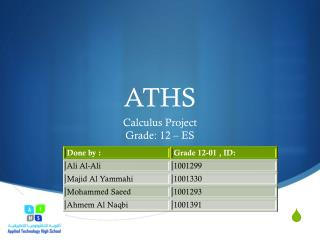DownloadDownload PresentationATHS

# ATHS

Download Presentation## ATHS

- - - - - - - - - - - - - - - - - - - - - - - - - - - E N D - - - - - - - - - - - - - - - - - - - - - - - - - - -
##### Presentation Transcript

1. ATHS Calculus Project Grade: 12 – ES

2. GOALS • Students will interpret their understanding of applications of integration • Students will use technology for research and communication: wiki/blog. • Students will present their findings to the class through digital Media(Video/Booklet).

3. Theme /INTRO • This project covers the application of topics in calculus; students will apply the concepts and theorems they have learned on integration. Moreover students will be able to apply their knowledge in relation to vari

4. TASK 1 • The boundaries of a piece of land that belongs to your family can be best described as in the following graph: • i.e. the area between the 𝑥−axis and the graph of the function 𝑓(𝑥)=𝑥3 − 5𝑥2 +6𝑥+5

5. Answer the following questions: • 1. Using approximating rectangles dividing the interval from 0 to 4 into 5 equal subdivisions, estimate the area between f(𝑥)= 𝑥3 − 5𝑥2 +6𝑥+5 and the 𝑥−axis, with ∆x=0.8 .

6. 2. Calculate the exact value of the same area using the definite integral.

7. Task 2”Oil Consumption” • Suppose that the rate of consumption of a natural resource is 𝑐′(𝑡), where: 𝑐′(𝑡) = 𝑘𝑒𝑟𝑡. • Here 𝑡 is time in years, 𝑟 is a constant, and 𝑘 is the consumption in the year when 𝑡 = 0. In 2006, an oil company sold 1.2 billion barrels of oil. Assume that 𝑟=0.04.

8. b) Set up a definite integral for the amount of oil that the company will sell in the next 10 years.

9. c) Evaluate the definite integral of part b.

10. d) The company has about 20 billions barrels of oil in reserve. To find the number of years that this amount will last, solve the equation:

11. e) Rework part d, assuming that 𝑟 = 0.02

12. Task 3 • The annual revenue for your company over the past five years was found to follow the following Integral formula: Where n is the number of years

13. 1. Verify the following relation between two consecutive years' revenue:

14. 2. Find the revenue of year 2, i.e. Find U2

15. Task 4 • A water well of depth 50 feet is used to supply people with water. A rope of weight 0.2 lbs/ft is used take the water out of the well using a barrel that weight 80 lbs when it is full of water.

16. a) Calculate the work needed to lift the barrel from the bottom to the midpoint of the well.

17. b) Calculate the work needed to lift the barrel from the midpoint of the well to the top of the well.